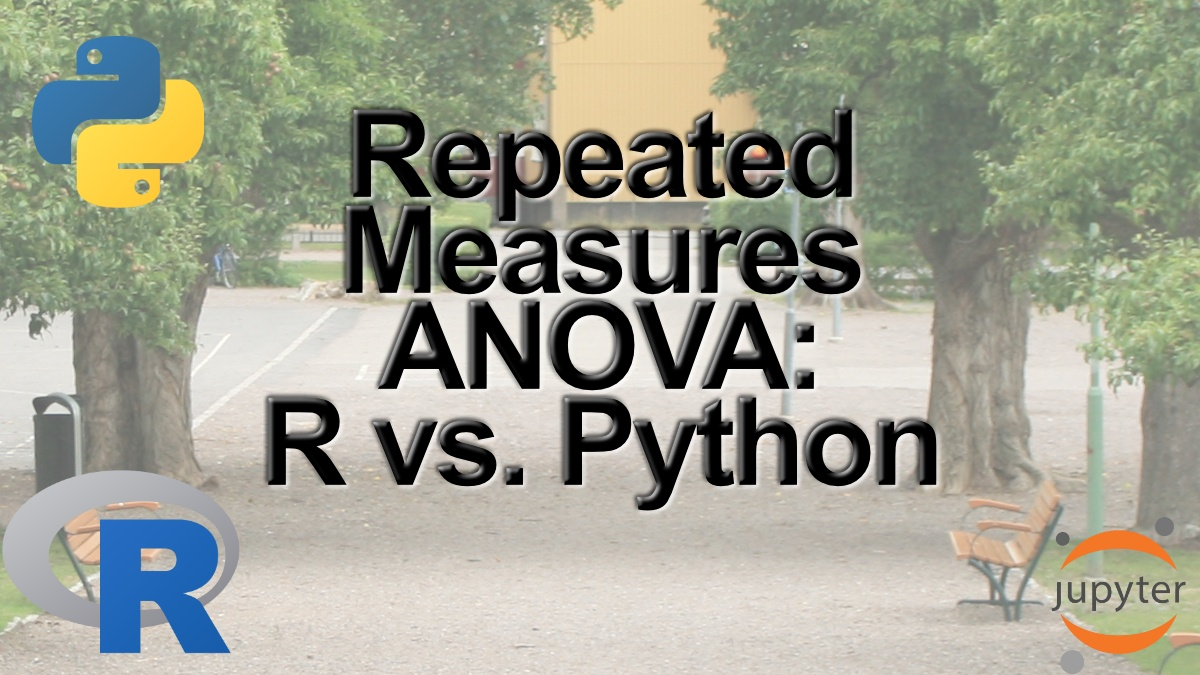## Repeated Measures ANOVA in R and Python using afex & pingouin

In this post, we will learn how to carry out repeated measures Analysis of Variance (ANOVA) in R and Python. To be specific, we will use the R package afex and the Python package pingouin to carry out one-way and two-way ANOVA for within-subject’s design. The...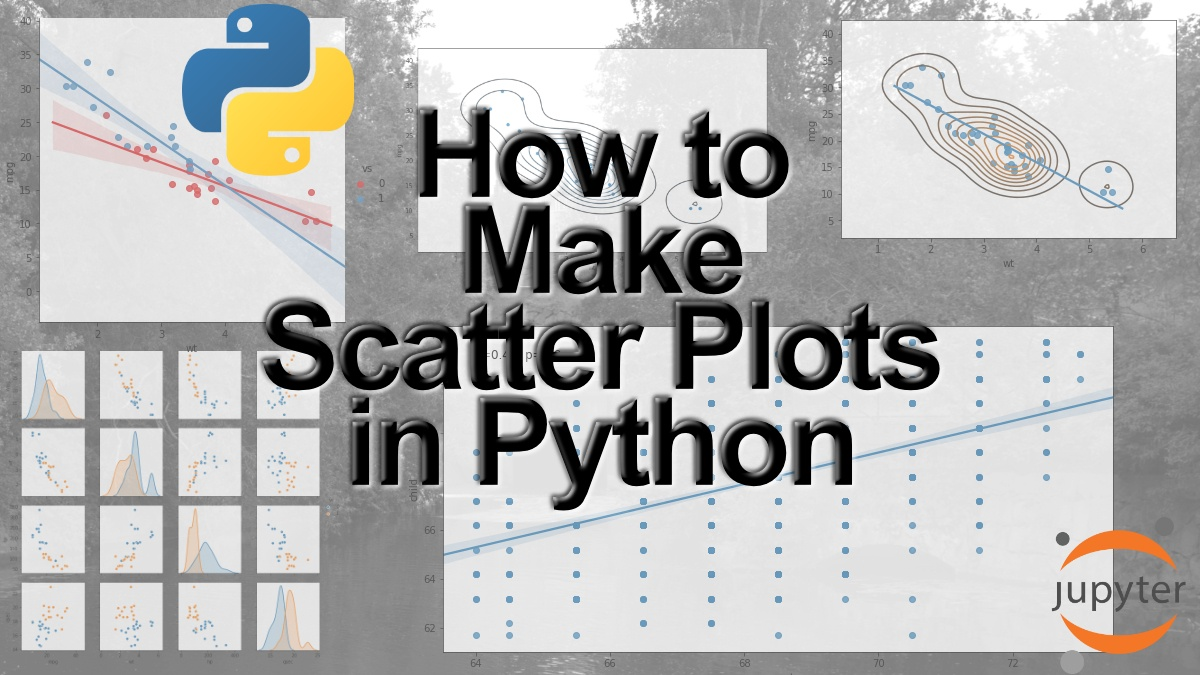## How to Make a Scatter Plot in Python using Seaborn

Data visualization is a big part of the process of data analysis. In this post, we will learn how to make a scatter plot using Python and the package Seaborn. In detail, we will learn how to use the Seaborn methods scatterplot, regplot, lmplot, and pairplot to create...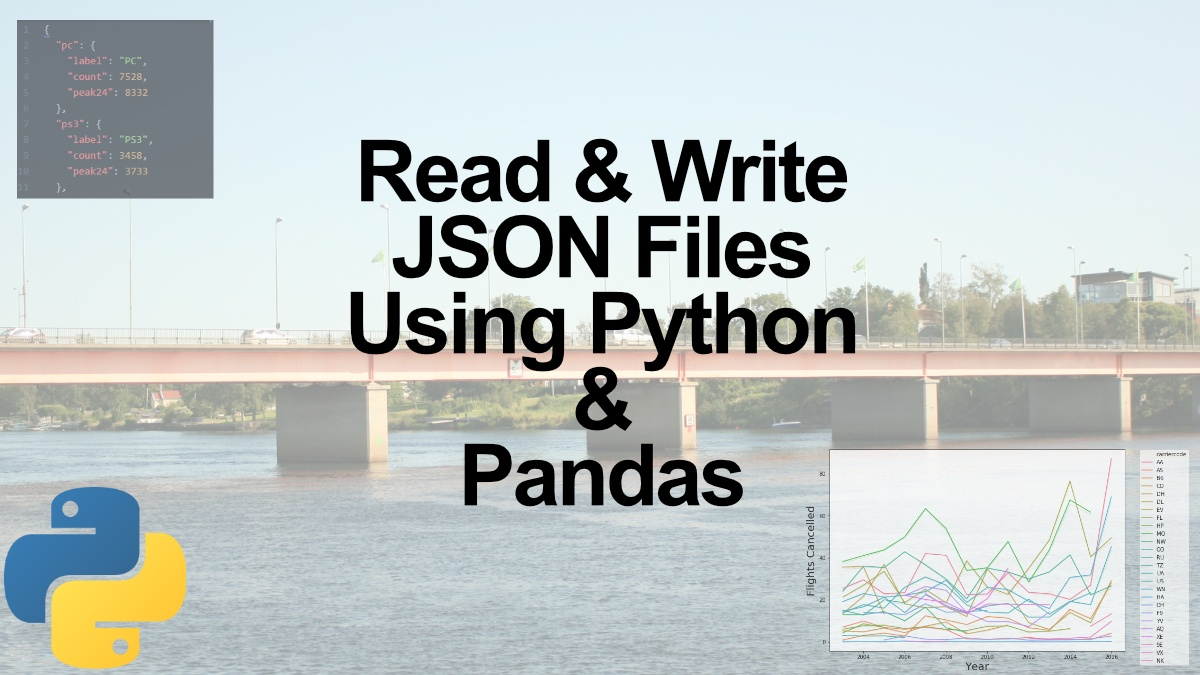## How to Read and Write JSON Files using Python and Pandas

In this post, we will learn how to read and write JSON files using Python. In the first part, we are going to use the Python package json to create and read a JSON file as well as write a JSON file. After that, we are going to use Pandas read_json method to load JSON...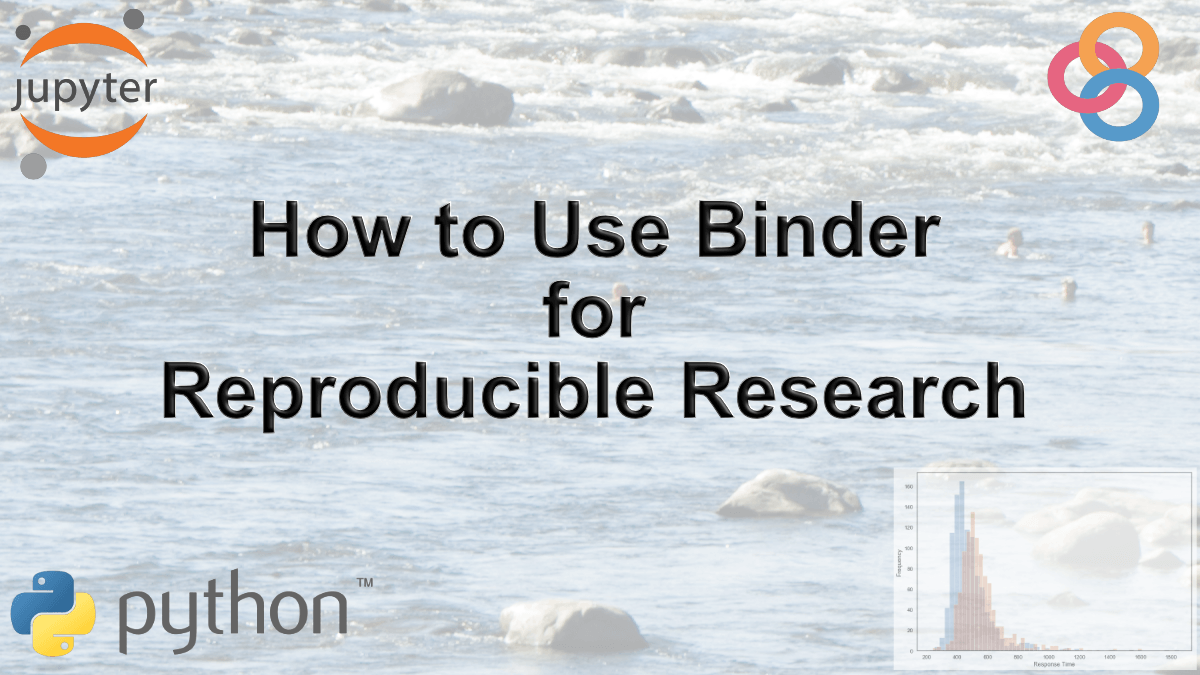## How to Use Binder and Python for Reproducible Research

In this post, we will learn how to create a binder so that our data analysis, for instance, can be fully reproduced by other researchers. That is, here we will learn how to use a tool called Binder for reproducible research. In previous posts, we have learned how to...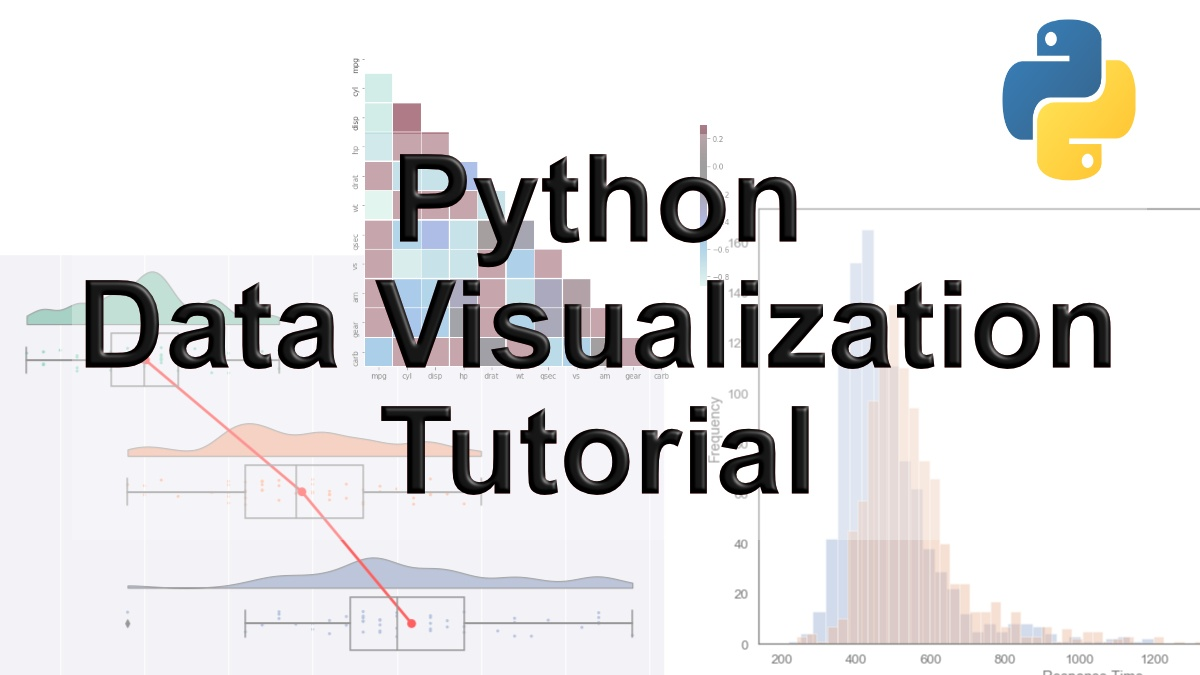## 9 Data Visualization Techniques You Should Learn in Python

With the ever-increasing volume of data, it is impossible to tell stories without visualizations. Data visualization is an art of how to turn numbers into useful knowledge. Using Python we can learn how to create data visualizations and present data in Python using...

## Probabilistic Programming in Python

Learn about probabilistic programming in this guest post by Osvaldo Martin, a researcher at The National Scientific and Technical Research Council of Argentina (CONICET) and author of Bayesian Analysis with Python: Introduction to statistical modeling and...